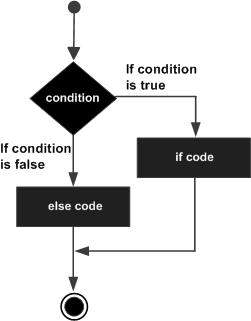# Tcl - If else Statement

An if statement can be followed by an optional else statement, which executes when the Boolean expression is false.

## Syntax

The syntax of an 'if...else' statement in Tcl language is −

```if {boolean_expression} {
# statement(s) will execute if the boolean expression is true
} else {
# statement(s) will execute if the boolean expression is false
}
```

If the Boolean expression evaluates to true, then the if block of code will be executed, otherwise else block of code will be executed.

Tcl language uses the expr command internally and hence it's not required for us to use expr statement explicitly.

### Flow Diagram## Example

```#!/usr/bin/tclsh

set a 100

#check the boolean condition
if {\$a < 20 } {
#if condition is true then print the following
puts "a is less than 20"
} else {
#if condition is false then print the following
puts "a is not less than 20"
}
puts "value of a is : \$a"
```

When the above code is compiled and executed, it produces the following result −

```a is not less than 20;
value of a is : 100
```

## The if...else if...else Statement

An 'if' statement can be followed by an optional else if...else statement, which is very useful to test various conditions using single if...else if statement.

When using if , else if, else statements there are few points to keep in mind −

• An 'if' can have zero or one else's and it must come after any else if's.

• An 'if' can have zero to many else if's and they must come before the else.

• Once an 'else if' succeeds, none of the remaining else if's or else's will be tested.

### Syntax

The syntax of an 'if...else if...else' statement in Tcl language is −

```if {boolean_expression 1} {
# Executes when the boolean expression 1 is true
} elseif {boolean_expression 2} {
# Executes when the boolean expression 2 is true
} elseif {boolean_expression 3} {
# Executes when the boolean expression 3 is true
} else {
# executes when the none of the above condition is true
}
```

### Example

```#!/usr/bin/tclsh

set a 100

#check the boolean condition
if { \$a == 10 } {
# if condition is true then print the following
puts "Value of a is 10"
} elseif { \$a == 20 } {
# if else if condition is true
puts "Value of a is 20"
} elseif { \$a == 30 } {
# if else if condition is true
puts "Value of a is 30"
} else {
# if none of the conditions is true
puts "None of the values is matching"
}

puts "Exact value of a is: \$a"
```

When the above code is compiled and executed, it produces the following result −

```None of the values is matching
Exact value of a is: 100
```
tcl_decisions.htm